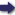(London :  Kegan Paul, Trench, Trübner & Co.,  1910.)

 Tools

## Search this bookPrev Page 44 Next``` 44 ALBERUNTS INDIA. Application of the rule to the gauge-year. Rule for the same purpose given by Ya'kub Ibn Tarik. Explanation of the latter method. Page 225. our gauge-year are 720,635,951,963. This number is given, and what we want to find is, how many Indian years and months are equal to this sum of days. Firstj we multiply the number by 55,739, and divide the prod uct by 3,506,481. The quotient is 11,45 5,2 24,5 7 5 iXnardtra days. We add this number to the civil days. The sum is 732,091,176,538 lunar days. We multiply them by 5 311, and divide the product by 178,111. The quotient is the number of ctdhimdsct days, viz. 21,829,849,018. We subtract them from the lunar days and get the remainder of 710,261,327,520, i.e. partial solar days. We divide these by 30 and get the quotient of 23,675,377,584, i.e. solar months. Dividing them by 12, we get Indian years, viz. 1,972,948,132, the same number of years of which our gauge-date consists, as we have already mentioned in a previous passage. Yakub Ibn Tarik has a note to the same effect: " Multiply the given civil days by the universal lunar days and divide the product by the universal civil days. Write down the quotient in two different places. In the one place multiply the number by the universal adhimdsct days and divide the product by the universal lunar days. The quotient gives the adhimdsa months. Multiply them by 30 and subtract the product from the number in the other place. The remainder is the number of partial solar days. You further reduce them to months and years." The rationale of this calculation is the following:— We have already mentioned that the given number of days are the difference between the lunar days and their unctrdtra, as the universal civil days are the dif¬ ference between the universal lunar days and their universal Unardtrct. These two measures stand in a constant relation to each other. Therefore we get the partial lunar days which are marked in two different places. Now, these are equal to the sum of the solar ```Prev Page 44 Next